A long-distance quantum key distribution scheme based on pre-detection of optical pulse with auxiliary state*
Quan Dong-Xiao†, Zhu Chang-Hua, Liu Shi-Quan, Pei Chang-Xing
State Key Laboratory of Integrated Services Networks, Xidian University, Xi’an 710071, China

Corresponding author. E-mail: dxquan@xidian.edu.cn

*Project supported by the National Natural Science Foundation of China (Grant No. 61372076), the Programme of Introducing Talents of Discipline to Universities, China (Grant No. B08038), and the Fundamental Research Funds for the Central Universities, China (Grant No. K5051201021).

Abstract

We construct a circuit based on PBS and CNOT gates, which can be used to determine whether the input pulse is empty or not according to the detection result of the auxiliary state, while the input state will not be changed. The circuit can be treated as a pre-detection device. Equipping the pre-detection device in the front of the receiver of the quantum key distribution (QKD) can reduce the influence of the dark count of the detector, hence increasing the secure communication distance significantly. Simulation results show that the secure communication distance can reach 516 km and 479 km for QKD with perfect single photon source and decoy-state QKD with weak coherent photon source, respectively.

Keyword: 03.67.Hk; 03.67.Dd; 03.67.Ac; quantum key distribution; pre-detection; secure communication distance; decoy state
1. Introduction

Quantum key distribution (QKD) provides a means of distributing secure keys between two remote parties, typically called Alice and Bob. In principle, QKD offers unconditional security guaranteed by the laws of physics. Unfortunately, there is a big gap between the theory and practice of QKD. In the practical QKD system, the imperfection of the setups will leave some loopholes for Eve to spy on the secret key. In fact, some potential quantum attack strategies have been discovered by exploiting the imperfection of the sources and the single photon detectors, such as the PNS attack, Trojan horse attack, time-shift attack, blinding attack and so on.

In 2003, Hwang proposed a fundamental primitive decoy state QKD protocol against PNS attack, in which the yield of the single photon pulse can be estimated precisely by the transmission rates of different intensity sources, hence the key generation rate and the communication distance are both increased significantly. In 2004, Wang proposed a scheme to implement the decoy state QKD in practical quantum cryptography. Ma et al. also researched on the decoy state QKD and put forward a scheme in which the quantum key generation rate can reach the performance of infinite intensity sources with only three different intensity sources. Then, a series of decoy state QKD protocols have been proposed. Decoy state is also used in the QKD protocol with heralded single-photon source and in the measurement device independent (MDI) QKD.

Another important field of quantum communication is quantum secure direct communication (QSDC), whose object is to transmit the secret messages directly without establishing a key in advance to encrypt them. In 2002, Bostrom and Felbinger proposed a “ ping-pong” QSDC protocol using entanglement. In 2003, Wojcik present an eavesdropping strategy that was based on the Ping-Pong protocol,  which reduces the efficiency to 50 percent while introducing no error in the control mode. In 2013, Pavicic made a modification of Wojcik’ s attack,  ingenious unitary operations were performed on the travel photons to tell Bell states without introducing errors in the information mode.

In this paper, based on the circuit presented in Ref. , an extra modification is made to simplify the implementation of the operation. Moreover, this device is used to detect whether or not the input pulse is empty, and direct measurement is not performed when there is no more than one photon in the input state. Equipping this device at the receiver of QKD can reduce the impact of the dark count rate of the detector, hence increasing the communication distance.

The rest of this paper is organized as follows. A modification of the device is made in Section 2, and a simple analysis on detecting whether the pulse is empty or with a photon is also given. In Section 3, the modified device is equipped at the receiver of QKD with perfect single photon source (PSPS) to decrease the error induced by the dark count of the detector, therefore increasing the communication distance. Section 4 discusses the circumstance in which this device is used at the receiver of decoy state QKD with a weak coherent photon source (WCPS). Finally, some conclusions are drawn in Section 5.

2. Description of the device

This section describes a device which can be used to determine whether or not the input pulse is empty, and direct measurement is not performed when there is no more than one photon in the input state. A schematic diagram of the device is shown in Fig. 1. An ingenious unitary operation composed of two Hadamard gates, a PBS gate, and two CNOT gates is denoted as Q. The PBS gate lets the horizontally polarized photons through and reflects the vertically polarized ones. The half-wave plate (HWP) flips the polarization of the photon HV, which is mathematically written as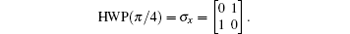Two auxiliary modes (x and y) with only one photon in the state | vac⟩ x| Hy are used in this article, where | vac⟩ x denotes the x mode is empty.

 Figure Option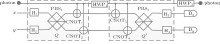Fig. 1. Schematic diagram of the device used to determine whether or not the pulse is empty.

Suppose that the state of the input photon isThe initial state of the whole system can be expressed as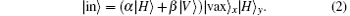Upon applying the first two Hadamard gates H1 and H2, on x and y modes, the state of the whole system will read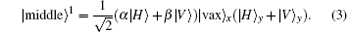After this point, two CNOT gates exist in the Pavicic Mladen’ s scheme,  but considering that the state of the control photon will not influence the x and y modes, these two CNOT gates can be omitted. The same reason goes for Q. The PBS acting on the x and y modes, performs the following transformation: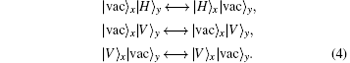The whole system after the PBS1 can then be expressed as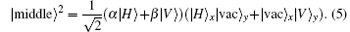A CNOT gate has two inputs: the control bit and the target bit. When the control bit is 1, the target bit will be flipped, or the target bit will remain unchanged. The whole system after the CNOT1 and CNOT2 under the control of the input photon reads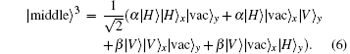The operator HWP1 yields the following state: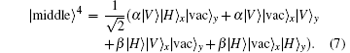Similar to the analysis above, after the whole system passes through Q, we obtain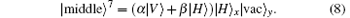Applying HWP2 on the input photon yields the final state of the whole system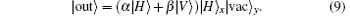If there is no photon in the pulse, then the initial state of the whole system is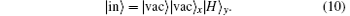The final state of the whole system is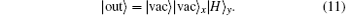According to Eqs. (9) and (11), if a photon exists in the pulse, then there will be a detection in the x mode; if the pulse is empty, the y detector will click. The state of the input photon is not changed. In fact, this device can be seen as a non-demolition measurement device, from the detection result of the x and y modes, single-photon pulse can be distinguished from vacuum pulses. This can be used to improve the performance of the QKD protocol.

3. Equipping in QKD with PSPS

For long-distance QKD, the error mainly induced by dark count of the detector generally reaches about 0.5, thus secure key cannot be obtained. Considering the scenario that the device shown in Fig. 1 is equipped in the front of general detection setups, pulses arriving at the receiver will be used as the control mode of the device before going through the following detection setups. A click of the x pre-detector means that the following main detection result is caused by a photon with great probability, thus it is valid. Otherwise, a click of the y pre-detector means that the following main detection result is generally caused by a dark count, and it will definitely be deleted. With the help of pre-detection, only the valid detection result will be chosen, hence reducing the error rate.

Suppose that the communication distance is L, the transmission probability of the channel is defined as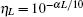, where the attenuation of fiber is described as α = 0.2 dB/ km. The probability a photon only cause a count in the x mode pre-detector is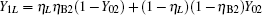where η B2 and Y02 represent the detect efficiency and dark count rate of the pre-detector, respectively, and accordingly η B and Y0, respectively, represent the detect efficiency and dark count rate of the following main detector. The yield rate of every pulse of the whole system can be written as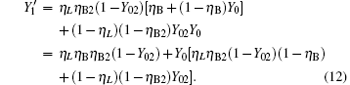Let ed represent the total misalignment error rate, the error rate of the whole system can be written as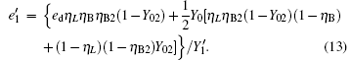The yield rate and error rate of the original system reads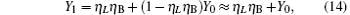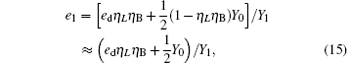compared with η B2, Y02 is very small, so we have η B2(1− Y02) ≈ η B2. For long-distance communication, η L will be very small, thus obtaining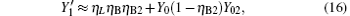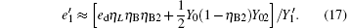Compared with Eq. (14), equation (16) can be understood as the transmission probability that the single photon is attenuated by η B2, the dark count rate is attenuated by (1 − η B2)Y02, so the yield of the source is decreased. In Eq. (15), at the communication distance when η Lη B = Y0, since ed ≪ 0.5, so the error is mainly induced by the dark count of the detector, the error rate is 25% ; with the distance increasing, η Lη B will be much smaller than Y0, then the error rate will reach about 0.5, apparently the secure key cannot be obtained. While in Eq. (17), at the communication distance when η Lη Bη B2 = Y0(1 − η B2)Y02 the error rate will be 0.25, this communication distance of QKD is much larger than that of the original scheme. In this way, the effective communication distance of QKD can be increased greatly, this will be proved in the following simulation.

The final key generation rate can be calculated by the GLLP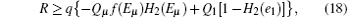where q = 1/ 2, H2(x) = − xlog2(x) − (1 − x) log2(1 − x), f(x) denotes the efficiency of two-way error correction. Due to the perfect single photon source, so Qμ = Q1 = Y1, Eμ = e1. Submitting Eqs. (12) and (13) into Eq. (18), we can get the key generation rate as the function of distance. The simulation parameters are selected coinciding with Ref. , they are ed = 0.033, η B = 0.045, Y0 = 1.7 × 10− 6, Y02 = 1.7 × 10− 6. The error rates of the sift key for the original QKD and the improved QKD proposed here are shown in Fig. 2. The final key generation rates for those two schemes are shown in Fig. 3.

 Figure Option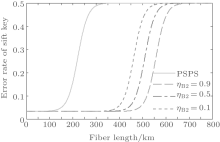Fig. 2. The error rate of the sift key for different circumstances.

 Figure Option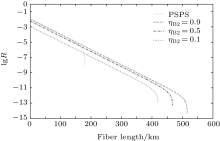Fig. 3. The final key generation rate for different circumstances.

Seen from Fig. 3, due to the pre-detection of the x mode, the final key generation rate is decreased, so the proposed scheme will not be suitable for a quantum communication whose distance is shorter than 160 km. From Fig. 2, we can see that the error rate is improved dramatically at a longer distance. Accordingly the communication distance will be increased significantly, as shown in Fig. 3, the improved QKD scheme with pre-detection mechanism can reach a communication distance of 516 km.

4. Equipping in decoy state QKD with WCPS

In the practical QKD, a WCPS source is generally adopted. Suppose the intensity of the source is μ , the number of photons existing in each pulse follows Poisson distribution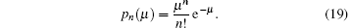The yield of the pulse with no photon is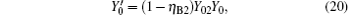and equation (12) represents the yield of the pulse with only one photon. For a multi-photon pulse, probably more than one photon will arrive at the pre-detection device, there will be a detection in the x mode or y mode with certain probability. According to Eq. (18), in order to get the lower bound of R, the lower bound of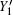and the upper bound of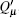,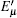, and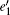should first be determined. Let η = η Lη B, for the original QKD scheme when Alice sends a pulse of n photon, the probability that at least one photon causes a count at Bob’ s detector is η n = 1 − (1 − η )n. To calculate the yield of the n-photon pulse, first consider the case that the arriving multi-photon pulse causes a count in the pre-detector of x mode, the upper bound of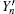and the lower bound of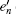can be written as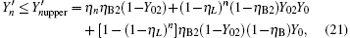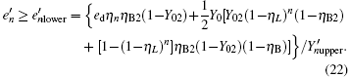In the other case assume that the arriving multi-photon pulse cause a count in the y mode of the pre-detector, we can then get the lower bound of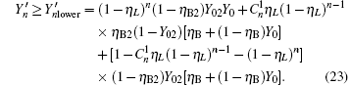The difference between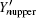and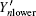is the processing of the multi-photon pulse: when the communication distance is long, the probability of a multi-photon arriving at the pre-detection device can be neglected because they will be the same as each other. The transmission probability of each pulse is given by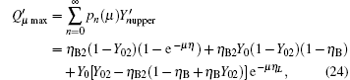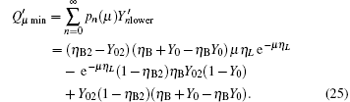The maximum error rate of each pulse can be written as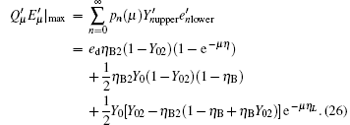It is well known that decoy states can be used to improve the performance of QKD obviously. Based on this, we further analyse the performance of the decoy-state QKD with a pre-detection mechanism. A three-state protocol is utilized here, suppose that the intensity of three sources is μ 1, μ 2 and 0, respectively, and μ 1 > μ 2. The source with vacuum states is used to estimate the dark count rate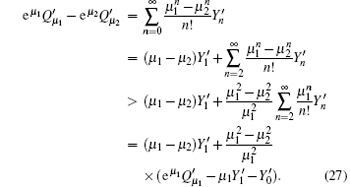We can obtain the lower bound of the yield of a single photon pulse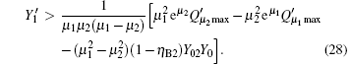The gain of the single photon pulse is given by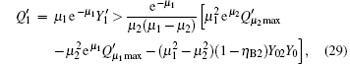and the upper bound of the error rate can be estimated by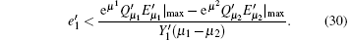Then substituting,,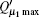, and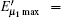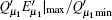into GLLP to simulate the performance of the three-state QKD protocol with pre-detection mechanism. In the simulation, the yield of the single-photon pulse is supposed to be estimated accurately, we can then get the optimal intensity of the signal source. Based on the optimal intensity of the signal source, the optimal intensity of the decoy state and the key generation rate can be obtained in turn.

 Figure Option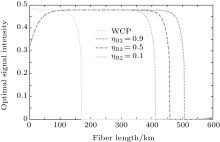Fig. 4. The optimal signal intensity for different circumstances.

 Figure Option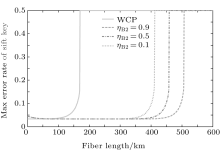Fig. 5. The max error rate of the sift key for different circumstances.

 Figure Option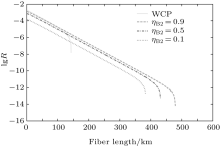Fig. 6. The final key generation rate for different circumstances.

The optimal signal intensity, the maximum error rate of the sift key, and the final key generation rate are shown in Figs. 4– 6, respectively. The green solid line shows the performance of the original decoy state QKD. The simulation results show that at the small communication distance, the Maximum error rate is a little bigger than that of the original QKD, because we give a loose estimate of the error come from multi-photon pulse. Thus, the optimal intensity is also lower to decrease the probability of multi-photon. Also, the final key generation rate is decreased due to the pre-detection of the x mode. The influence of multi-photon is decreased with the increase of the communication distance, the advantage of pre-detection is visible, the error rate is reduced dramatically, and the security distance can reach 479 km.

5. Conclusion

In this paper, we construct a circuit with PBS and CNOT gates, which can be used to determine whether or not the input pulse is empty according to the detection result of the auxiliary state, while the input state will not be changed. Equipping this device at the receiver can reduce the influence of the dark count of the detector for long-distance communication. Therefore, the secure communication distance can, respectively, reach 516 km and 479 km for QKD with PSPS and decoy-state QKD with WCPS, which has been shown in simulation results. When it comes to real-world implementation, synchronization of the signal with the auxiliary state and the main detector should be ensured, which can be realized with the help of the synchronization pulse in QKD protocol. The result of the main detector will be deleted if there is a corresponding detection in the y mode, the subsequent process is the same as QKD.

Reference
 1 Bennett C H and Brassard G 1984Proceedings of the IEEE International Conference on Computers, Systems, and Signal ProcessingBangalore, Indiap. 175 [Cited within:1] 2 Ekert A 1991 Phys. Rev. Lett. 67 661 DOI:10.1103/PhysRevLett.67.661 [Cited within:1] [JCR: 7.943] 3 Bennett C H, Brassard G and Mermin N D 1992 Phys. Rev. Lett. 68 557 DOI:10.1103/PhysRevLett.68.557 [Cited within:1] [JCR: 7.943] 4 LO H K and Chau H 1999 Science 283 2050 DOI:10.1126/science.283.5410.2050 [Cited within:1] 5 Shor P W and Preskill J 2000 Phys. Rev. Lett. 85 441 DOI:10.1103/PhysRevLett.85.441 [Cited within:1] [JCR: 7.943] 6 Gottesman D, Lo H K, Lutkenhaus N and Preskill J 2004 Quantum Inf. Comput. 4 325 [Cited within:2] [JCR: 1.646] 7 Hwang W Y 2003 Phys. Rev. Lett. 91 057901 DOI:10.1103/PhysRevLett.91.057901 [Cited within:1] [JCR: 7.943] 8 Wang X B 2005 Phys. Rev. Lett. 94 230503 DOI:10.1103/PhysRevLett.94.230503 [Cited within:1] [JCR: 7.943] 9 Ma X F, Qi B, Zhao Y and Lo H K 2005 Phys. Rev. A 72 012326 DOI:10.1103/PhysRevA.72.012326 [Cited within:1] [JCR: 3.042] 10 Chi H H, Yu Z W and Wang X B 2012 Phys. Rev. A 86 042307 DOI:10.1103/PhysRevA.86.042307 [Cited within:1] [JCR: 3.042] 11 Piparo N L and Razavi M 2012 Phys. Rev. A 88 012332 DOI:10.1103/PhysRevA.88.012332 [Cited within:1] [JCR: 3.042] 12 Jiao R Z, Zhang C and Ma H Q 2011 Acta Phys. Sin. 60 110303(in Chinese) [Cited within:1] [JCR: 1.016] [CJCR: 1.691] 13 Xu F X, Wang S, Han Z F and Guo G C 2010 Chin. Phys. B 19 100312 DOI:10.1088/1674-1056/19/10/100312 [Cited within:1] [JCR: 1.148] [CJCR: 1.2429] 14 Wang Q, Wang X B, Björk G and Karlsson A 2007 EPL 79 40001 DOI:10.1209/0295-5075/79/40001 [Cited within:1] [JCR: 2.26] 15 Dong C, Zhao S H, Zhao W H, Shi L and Zhao G H 2014 Acta Phys. Sin. 63 030302(in Chinese) [Cited within:1] [JCR: 1.016] [CJCR: 1.691] 16 Yu Z W, Zhou Y H and Wang X B 2013 Phys. Rev. A 88 062339 DOI:10.1103/PhysRevA.88.062339 [Cited within:1] [JCR: 3.042] 17 Lo H K, Curty M and Qi B 2012 Phys. Rev. Lett. 108 130503 DOI:10.1103/PhysRevLett.108.130503 [Cited within:2] [JCR: 7.943] 18 Bostrom K and Felbinger T 2002 Phys. Rev. Lett. 89 187902 DOI:10.1103/PhysRevLett.89.187902 [Cited within:1] [JCR: 7.943] 19 Wojcik A 2003 Phys. Rev. Lett. 90 157901 DOI:10.1103/PhysRevLett.90.157901 [Cited within:1] [JCR: 7.943] 20 Mladen P 2013 Phys. Rev. A 87 042326 DOI:10.1103/PhysRevA.87.042326 [Cited within:3] 21 Gobby C, Yuan Z L and Shields A J 2004 Appl. Phys. Lett. 84 3762 DOI:10.1063/1.1738173 [Cited within:1] [JCR: 3.794]